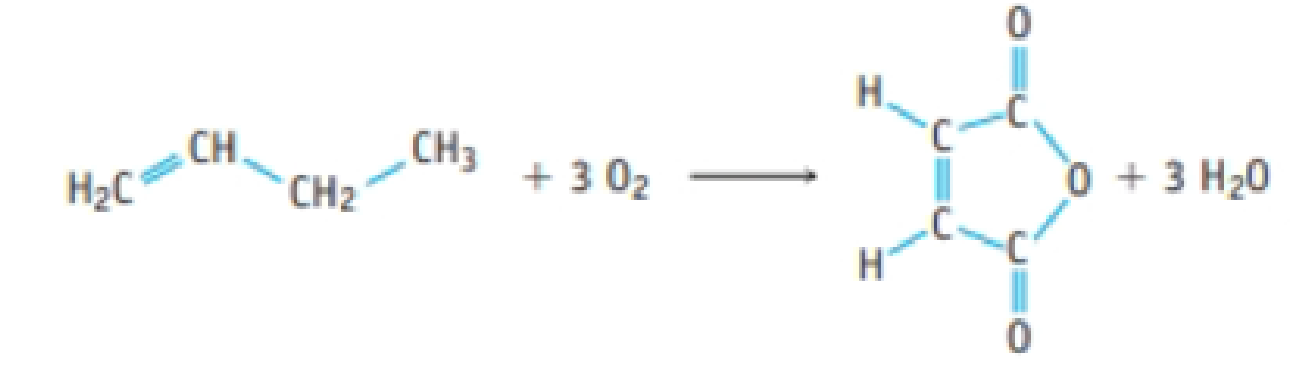# ATOM ECONOMY: Maleic anhydride, C 4 H 2 O 3 , can be produced by the oxidation of benzene (Study Question 141). It can also be produced from the oxidation of butene. (a) What is the % atom economy for the synthesis of maleic anhydride from butene by this reaction? (b) If 1.02 kg of maleic anhydride is produced from exactly 1.00 kg of butene, what is the percent yield of the anhydride? What mass of the by-product H 2 O is also produced?### Chemistry & Chemical Reactivity

9th Edition
John C. Kotz + 3 others
Publisher: Cengage Learning
ISBN: 9781133949640

#### Solutions

Chapter
Section### Chemistry & Chemical Reactivity

9th Edition
John C. Kotz + 3 others
Publisher: Cengage Learning
ISBN: 9781133949640
Chapter 4, Problem 142SCQ
Textbook Problem
439 views

## ATOM ECONOMY: Maleic anhydride, C4H2O3, can be produced by the oxidation of benzene (Study Question 141). It can also be produced from the oxidation of butene.(a) What is the % atom economy for the synthesis of maleic anhydride from butene by this reaction? (b) If 1.02 kg of maleic anhydride is produced from exactly 1.00 kg of butene, what is the percent yield of the anhydride? What mass of the by-product H2O is also produced?

a)

Interpretation Introduction

Interpretation:

The % atom economy for the product in the given reaction has to be calculated.

Concept introduction:

• Atom economy is one of the key parameter used to evaluate the efficiency of a reaction.

%atomeconomy=molarmassofatomutilizedmolarmassofreactants×100%

• Percent yield of reaction is the ratio of mass of actual yield to the mass of theoretical yield and multiplied with hundred.
• Numberofmole=GivenmassofthesubstanceMolarmass
• The molar mass of an element or compound is the mass in grams of 1 mole of that substance, and it is expressed in the unit of grams per mol (g/mol).
• For chemical reaction balanced chemical reaction equation written in accordance with the Law of conservation of mass.
• Law of conservation of mass states that for a reaction total mass of the reactant and product must be equal.
• Stoichiometric factor is a relationship between reactant and product which is obtained from the balanced chemical equation for a particular reaction.

### Explanation of Solution

Atom economy is one of the key parameter used to evaluate the efficiency of a reaction. Atom economy percentage of a reaction can be calculated by using the following equation.

%atomeconomy=molarmassofatomutilizedmolarmassofreactants×100%

The balanced equation for the synthesis of maleic anhydride from butene is,

In the above reaction reactant molecule contains 4C,8H,1O,and3O2 so the combined molar mass of the reactants is 152g/mol

The product molecule maleicanhydrideC4H2O3 contains 4C </

(b)

Interpretation Introduction

Interpretation:

The product in the given reaction, percentage yield and the mass of H2O produced in the reaction also has to be calculated.

Concept introduction:

• Atom economy is one of the key parameter used to evaluate the efficiency of a reaction.

%atomeconomy=molarmassofatomutilizedmolarmassofreactants×100%

• Percent yield of reaction is the ratio of mass of actual yield to the mass of theoretical yield and multiplied with hundred.
• Numberofmole=GivenmassofthesubstanceMolarmass
• The molar mass of an element or compound is the mass in grams of 1 mole of that substance, and it is expressed in the unit of grams per mol (g/mol).
• For chemical reaction balanced chemical reaction equation written in accordance with the Law of conservation of mass.
• Law of conservation of mass states that for a reaction total mass of the reactant and product must be equal.
• Stoichiometric factor is a relationship between reactant and product which is obtained from the balanced chemical equation for a particular reaction.

### Still sussing out bartleby?

Check out a sample textbook solution.

See a sample solution

#### The Solution to Your Study Problems

Bartleby provides explanations to thousands of textbook problems written by our experts, many with advanced degrees!

Get Started

Find more solutions based on key concepts
What is the mass of a 43.8-mL sample of gasoline, which has a density of 0.70 g/cm3?

General Chemistry - Standalone book (MindTap Course List)

Fried banana or vegetable snack chips make a healthy everyday snack choice for vegetarians. T F

Nutrition: Concepts and Controversies - Standalone book (MindTap Course List)

How many autosomes are present in a body cell of a human being? In a gamete?

Human Heredity: Principles and Issues (MindTap Course List)

25-39 What kind of functions do ribozymes, in general, perform?

Introduction to General, Organic and Biochemistry

What were the main stimuli to European voyages of exploration during the Age of Discovery?

Oceanography: An Invitation To Marine Science, Loose-leaf Versin

A long, straight wire carries a current I. A right-angle bend is made in the middle of the wire. The bend forms...

Physics for Scientists and Engineers, Technology Update (No access codes included)